# Is the right behind inlfation Gabriela Barenboim SILAFAE

• Slides: 42Is the right behind inlfation ? Gabriela Barenboim SILAFAE 09Unsolved issues in the standard model • Horizon problem Why is the CMB so smooth ? • The flatness problem Why is the Universe flat ? Why is Ω ~ 1 ? • The structure problem Where do the fluctuations in the CMB come from ? • The relic problem Why aren’t there magnetic monopoles ?Outstanding Problems • Why is the CMB so isotropic? – consider matter-only universe: • horizon distance d. H(t) = 3 ct • scale factor a(t) = (t/t 0)2/3 • therefore horizon expands faster than the universe – “new” objects constantly coming into view – CMB decouples at 1+z ~ 1000 • i. e. t. CMB = t 0/104. 5 • d. H(t. CMB) = 3 ct 0/104. 5 • now this has expanded by a factor of 1000 to 3 ct 0/101. 5 • but horizon distance now is 3 ct 0 • so angle subtended on sky by one CMB horizon distance is only 10− 1. 5 rad ~ 2° – patches of CMB sky >2° apart should not be causally connectedOutstanding Problems • Why is universe so flat? – a multi-component universe satisfies and, neglecting Λ, – therefore • during radiation dominated era |1 – Ω(t)| a 2 • during matter dominated era |1 – Ω(t)| a • if |1 – Ω 0| < 0. 06 (WMAP). . . then at CMB emission |1 – Ω| < 0. 00006 – we have a fine tuning problem!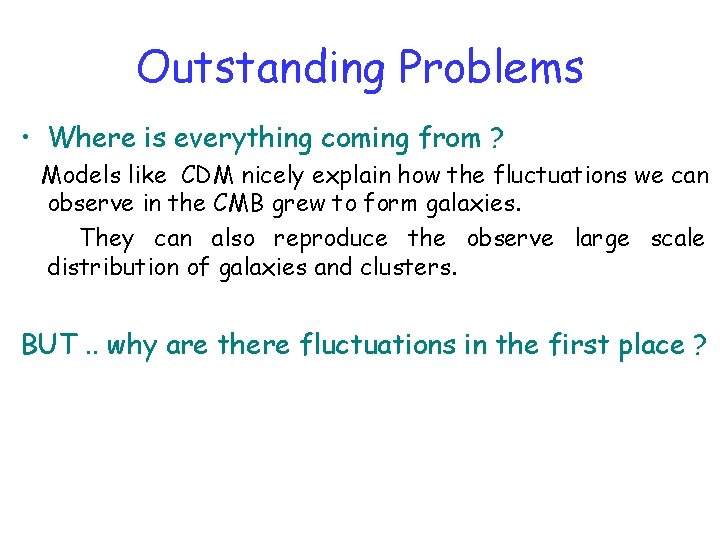Outstanding Problems • Where is everything coming from ? Models like CDM nicely explain how the fluctuations we can observe in the CMB grew to form galaxies. They can also reproduce the observe large scale distribution of galaxies and clusters. BUT. . why are there fluctuations in the first place ?Outstanding Problems • Where is everything coming from ?Outstanding Problems • Where is everything coming from ?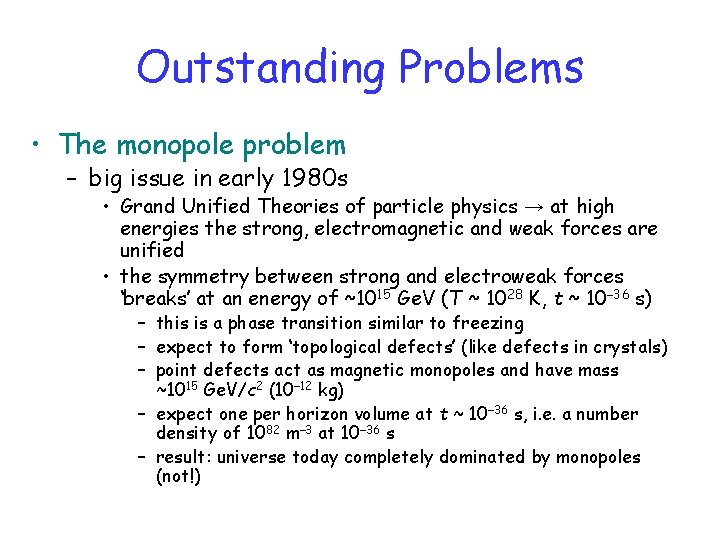Outstanding Problems • The monopole problem – big issue in early 1980 s • Grand Unified Theories of particle physics → at high energies the strong, electromagnetic and weak forces are unified • the symmetry between strong and electroweak forces ‘breaks’ at an energy of ~1015 Ge. V (T ~ 1028 K, t ~ 10− 36 s) – this is a phase transition similar to freezing – expect to form ‘topological defects’ (like defects in crystals) – point defects act as magnetic monopoles and have mass ~1015 Ge. V/c 2 (10− 12 kg) – expect one per horizon volume at t ~ 10− 36 s, i. e. a number density of 1082 m− 3 at 10− 36 s – result: universe today completely dominated by monopoles (not!)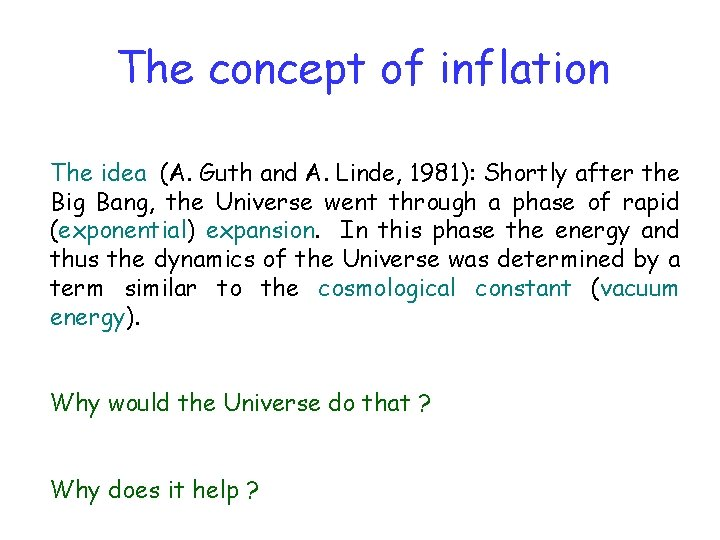The concept of inflation The idea (A. Guth and A. Linde, 1981): Shortly after the Big Bang, the Universe went through a phase of rapid (exponential) expansion. In this phase the energy and thus the dynamics of the Universe was determined by a term similar to the cosmological constant (vacuum energy). Why would the Universe do that ? Why does it help ?Inflation and the horizon • Assume large positive cosmological constant Λ acting from tinf to tend • then for tinf < tend a(t) = a(tinf) exp[Hi(t – tinf)] – Hi = (⅓ Λ)1/2 – if Λ large a can increase by many orders of magnitude in a very short time • Exponential inflation is the usual assumption but a power law a = ainf(t/tinf)n works if n > 1 with inflation horizon without inflation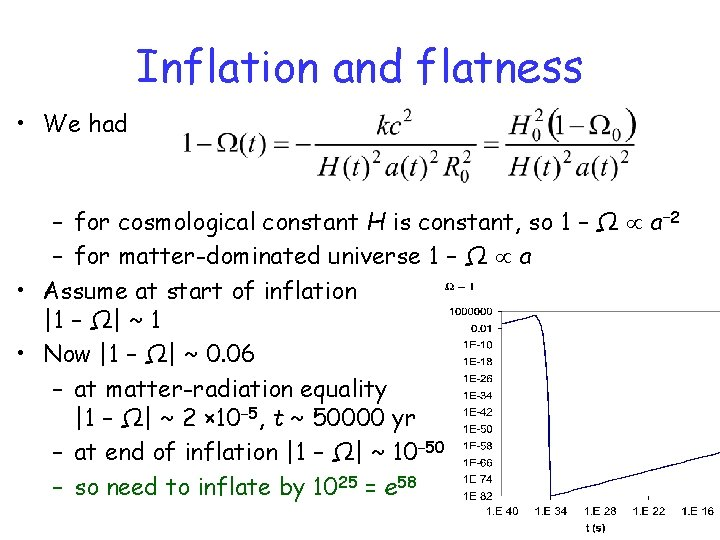Inflation and flatness • We had – for cosmological constant H is constant, so 1 – Ω a− 2 – for matter-dominated universe 1 – Ω a • Assume at start of inflation |1 – Ω| ~ 1 • Now |1 – Ω| ~ 0. 06 – at matter-radiation equality |1 – Ω| ~ 2 × 10− 5, t ~ 50000 yr – at end of inflation |1 – Ω| ~ 10− 50 – so need to inflate by 1025 = e 58Inflation and the structure problem • Before inflation: quantum fluctuations • Inflation amplifies quantum fluctuations to macroscopic scales • After inflation macroscopic fluctuations (as can be observed in the CMB radiation) provide the seeds from which galaxies form.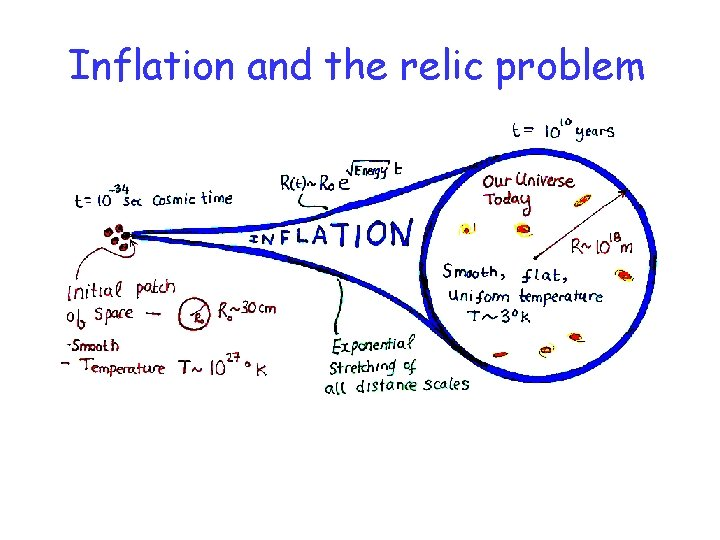Inflation and the relic problem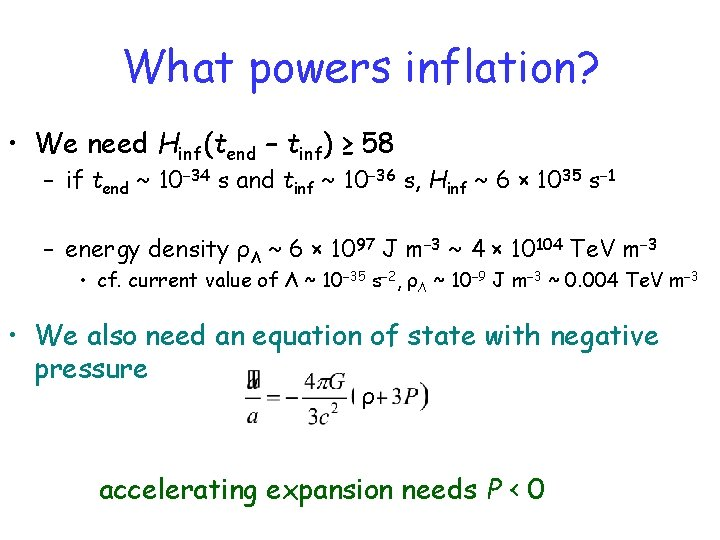What powers inflation? • We need Hinf(tend – tinf) ≥ 58 – if tend ~ 10− 34 s and tinf ~ 10− 36 s, Hinf ~ 6 × 1035 s− 1 – energy density ρΛ ~ 6 × 1097 J m− 3 ~ 4 × 10104 Te. V m− 3 • cf. current value of Λ ~ 10− 35 s− 2, ρΛ ~ 10− 9 J m− 3 ~ 0. 004 Te. V m− 3 • We also need an equation of state with negative pressure ρ accelerating expansion needs P < 0Inflation with scalar field • Need potential U with broad nearly flat plateau near φ = 0 – metastable false vacuum – inflation as φ moves very slowly away from 0 – stops at drop to minimum (true vacuum) • decay of inflaton field at this point reheats universe, producing photons, quarks etc. (but not monopoles – too heavy) • equivalent to latent heat of a phase transition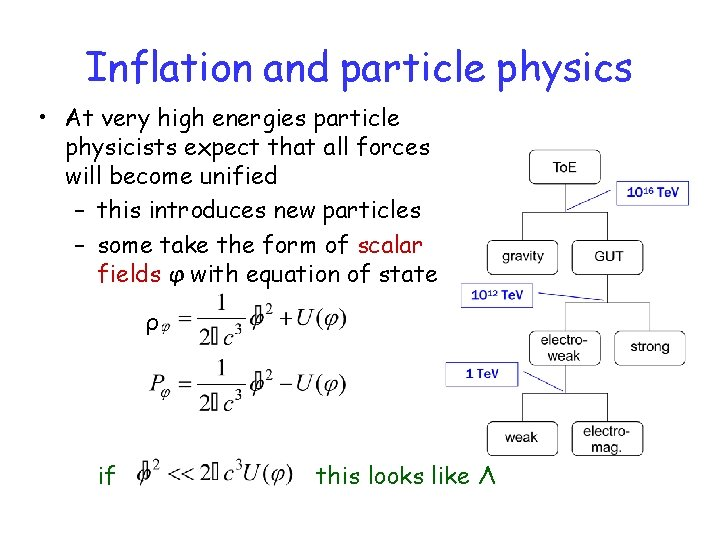Inflation and particle physics • At very high energies particle physicists expect that all forces will become unified – this introduces new particles – some take the form of scalar fields φ with equation of state ρ if this looks like Λ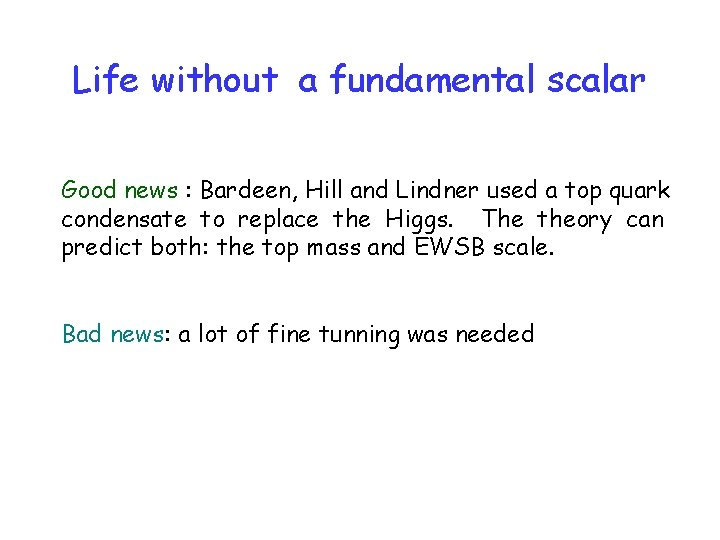Life without a fundamental scalar Good news : Bardeen, Hill and Lindner used a top quark condensate to replace the Higgs. The theory can predict both: the top mass and EWSB scale. Bad news: a lot of fine tunning was neededR R R Hitoshi Murayama B-L WS LBNL 18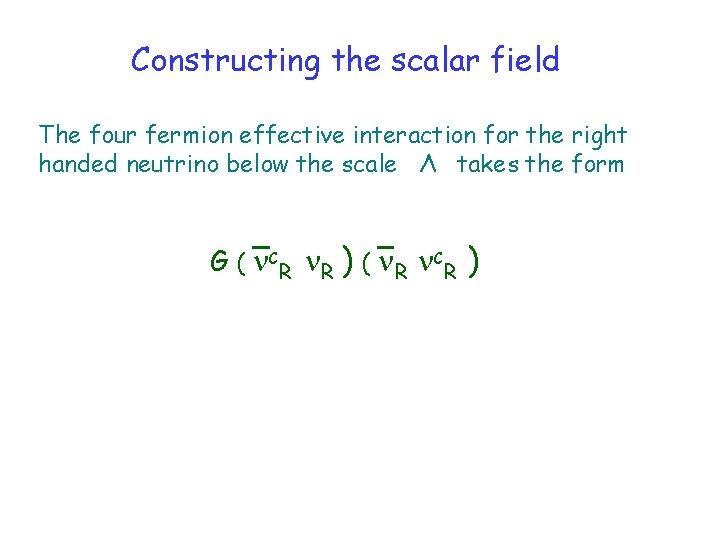Constructing the scalar field The four fermion effective interaction for the right handed neutrino below the scale Λ takes the form G ( CR R ) ( R CR )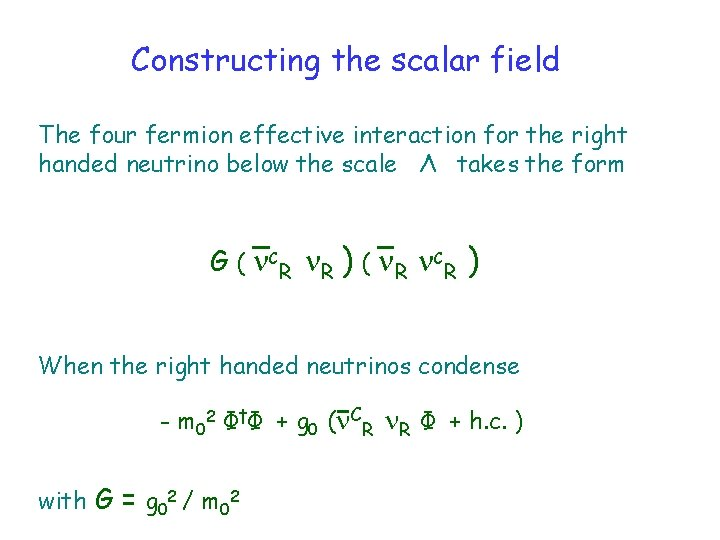Constructing the scalar field The four fermion effective interaction for the right handed neutrino below the scale Λ takes the form G ( CR R ) ( R CR ) When the right handed neutrinos condense - m 02 Φ†Φ + g 0 ( CR R Φ + h. c. ) with G = g 02 / m 02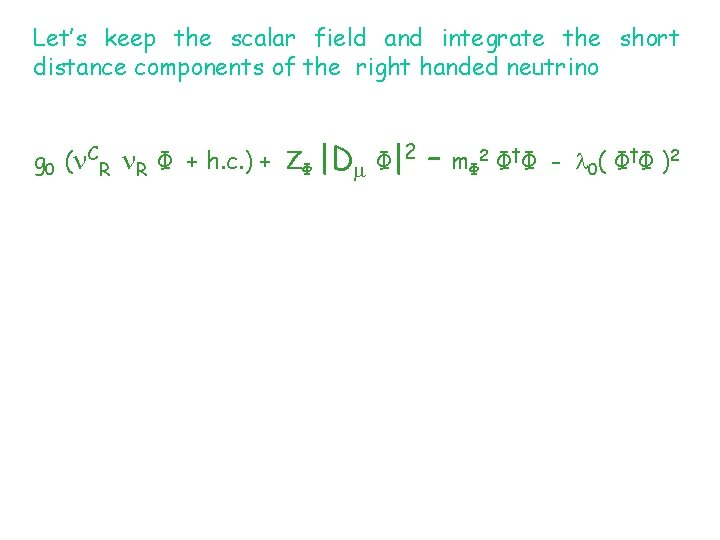Let’s keep the scalar field and integrate the short distance components of the right handed neutrino g 0 ( CR R Φ + h. c. ) + ZΦ |D Φ|2 – m Φ 2 Φ † Φ - 0 ( Φ † Φ ) 2Let’s keep the scalar field and integrate the short distance components of the right handed neutrino g 0 ( CR R Φ + h. c. ) + ZΦ |D Φ|2 – m Φ 2 Φ † Φ - 0 ( Φ † Φ ) 2 where ZΦ = Nf g 02 / (4 )2 ln (Λ 2 / 2 ) mΦ 2 = m 02 – 2 Nf g 02 / (4 )2 (Λ 2 - 2 ) 0 = Nf g 04 / (4 )2 ln (Λ 2 / 2 )Let’s keep the scalar field and integrate the short distance components of the right handed neutrino g 0 ( CR R Φ + h. c. ) + ZΦ |D Φ|2 – m Φ 2 Φ † Φ - 0 ( Φ † Φ ) 2 where ZΦ = Nf g 02 / (4 )2 ln (Λ 2 / 2 ) mΦ 2 = m 02 – 2 Nf g 02 / (4 )2 (Λ 2 - 2 ) 0 = Nf g 04 / (4 )2 ln (Λ 2 / 2 )Let’s keep the scalar field and integrate the short distance components of the right handed neutrino g 0 ( CR R Φ + h. c. ) + ZΦ |D Φ|2 – m Φ 2 Φ † Φ - 0 ( Φ † Φ ) 2 where ZΦ = Nf g 02 / (4 )2 ln (Λ 2 / 2 ) mΦ 2 = m 02 – 2 Nf g 02 / (4 )2 (Λ 2 - 2 ) 0 = Nf g 04 / (4 )2 ln (Λ 2 / 2 )Let’s keep the scalar field and integrate the short distance components of the right handed neutrino rescale the scalar field Φ → Φ / (ZΦ )1/2 g 0 ( CR R Φ + h. c. ) + ZΦ |D Φ|2 – m Φ 2 Φ † Φ - 0 ( Φ † Φ ) 2 where ZΦ = Nf g 02 / (4 )2 ln (Λ 2 / 2 ) mΦ 2 = m 02 – 2 Nf g 02 / (4 )2 (Λ 2 - 2 ) 0 = Nf g 04 / (4 )2 ln (Λ 2 / 2 )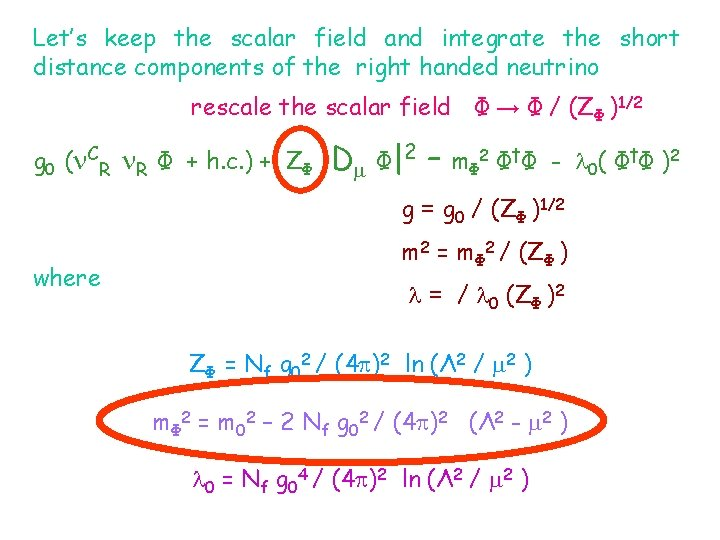Let’s keep the scalar field and integrate the short distance components of the right handed neutrino rescale the scalar field Φ → Φ / (ZΦ )1/2 g 0 ( CR R Φ + h. c. ) + ZΦ |D Φ|2 – m Φ 2 Φ † Φ - 0 ( Φ † Φ ) 2 g = g 0 / (ZΦ )1/2 where m 2 = mΦ 2 / (ZΦ ) = / 0 (ZΦ )2 ZΦ = Nf g 02 / (4 )2 ln (Λ 2 / 2 ) mΦ 2 = m 02 – 2 Nf g 02 / (4 )2 (Λ 2 - 2 ) 0 = Nf g 04 / (4 )2 ln (Λ 2 / 2 )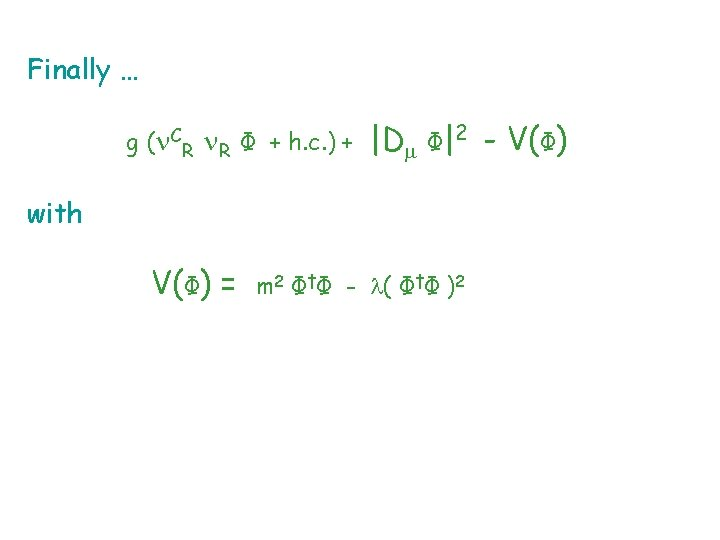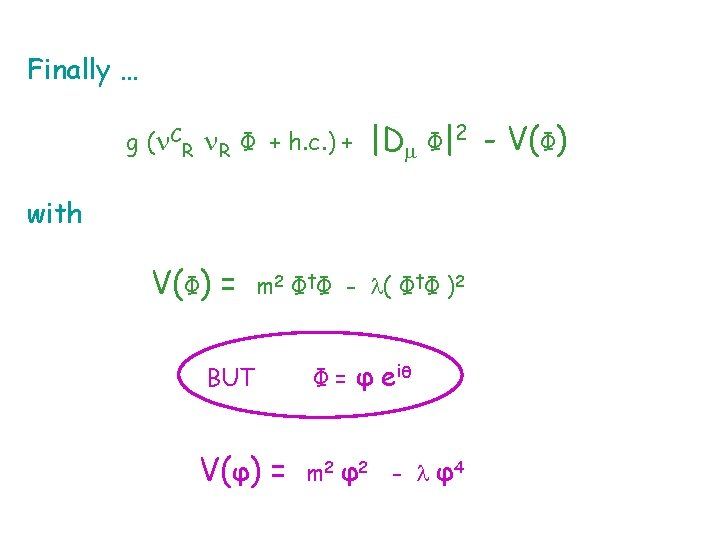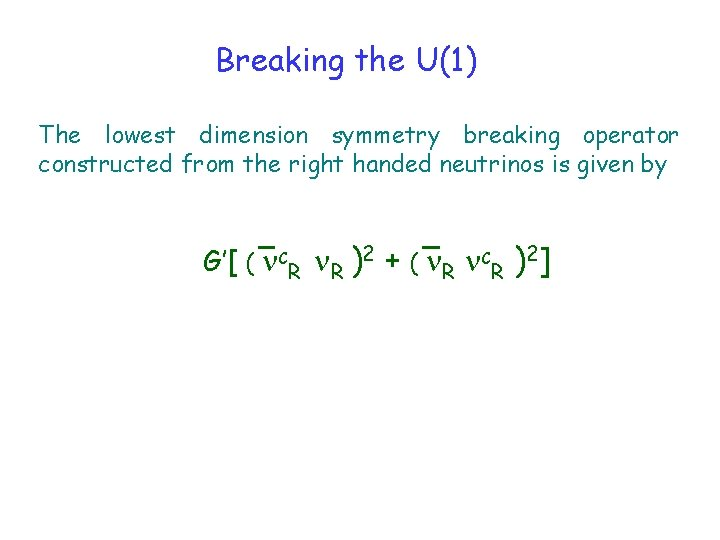Breaking the U(1) The lowest dimension symmetry breaking operator constructed from the right handed neutrinos is given by G’[ ( CR R )2 + ( R CR )2]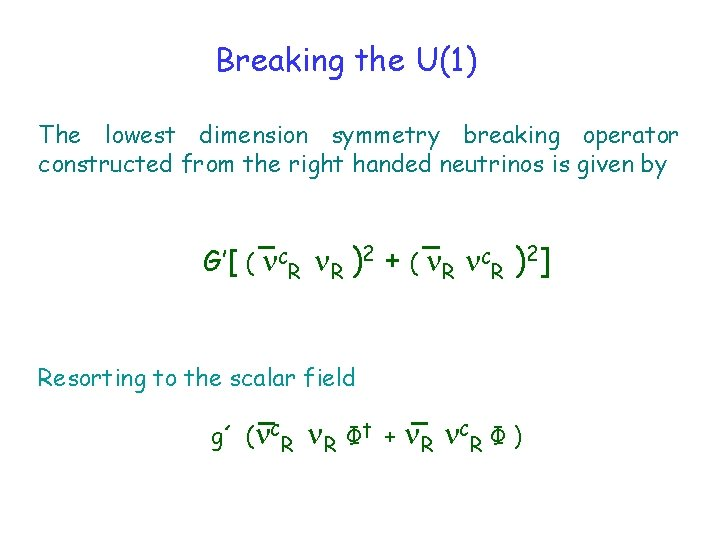Breaking the U(1) The lowest dimension symmetry breaking operator constructed from the right handed neutrinos is given by G’[ ( CR R )2 + ( R CR )2] Resorting to the scalar field g´ ( CR R Φ† + R CR Φ )at 1 -loop m. R 2(θ) = (g 2 + g´ 2 + 2 g g´ Cos(θ) ) v 2 V(θ)=- g 2 g’ 2 v 4 (16 2) [ ] [ g 2 + g’ 2 + Cos(θ) 2 gg’ 2 ln g 2 + g’ 2 + 2 gg´ Cos(θ) ]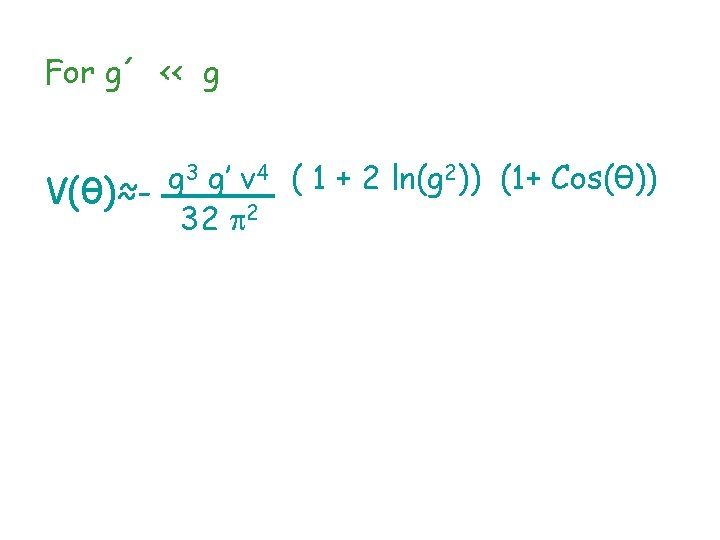For g´ ‹‹ g 3 g’ v 4 ( 1 + 2 ln(g 2)) (1+ Cos(θ)) g V(θ)≈32 2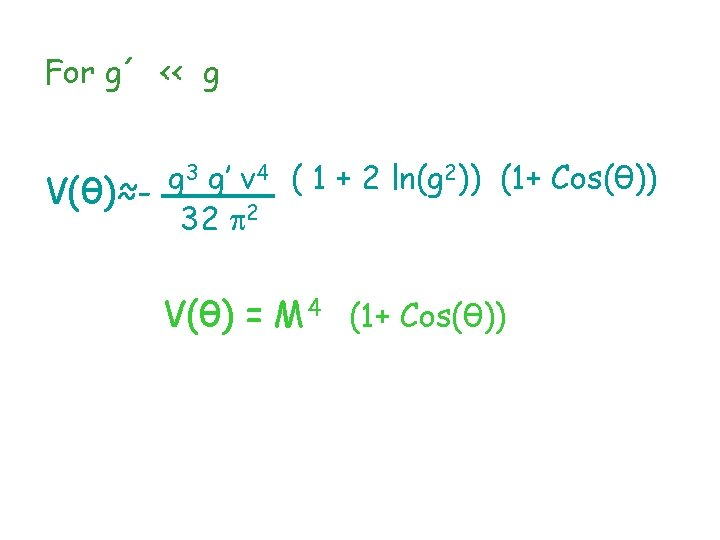For g´ ‹‹ g 3 g’ v 4 ( 1 + 2 ln(g 2)) (1+ Cos(θ)) g V(θ)≈32 2 V(θ) = M 4 (1+ Cos(θ))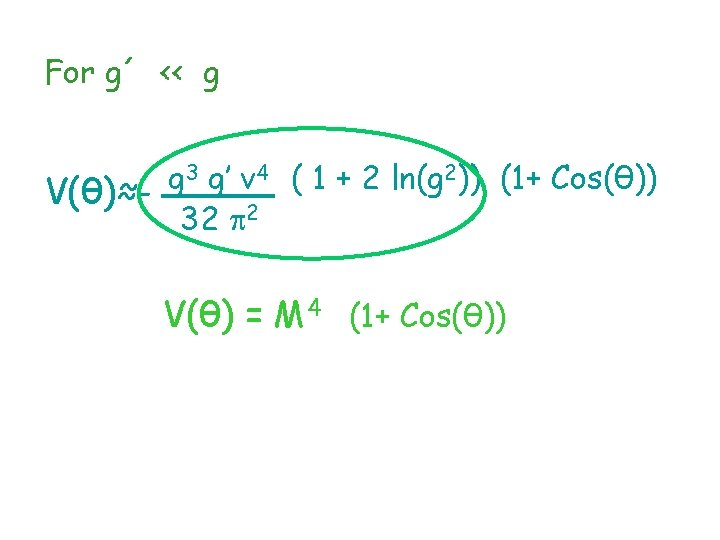For g´ ‹‹ g 3 g’ v 4 ( 1 + 2 ln(g 2)) (1+ Cos(θ)) g V(θ)≈32 2 V(θ) = M 4 (1+ Cos(θ))Inflation phenomenology ns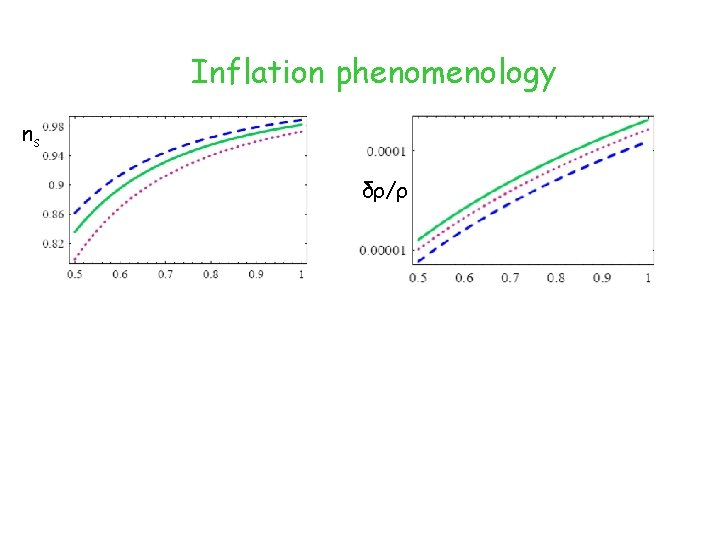Inflation phenomenology ns δρ/ρ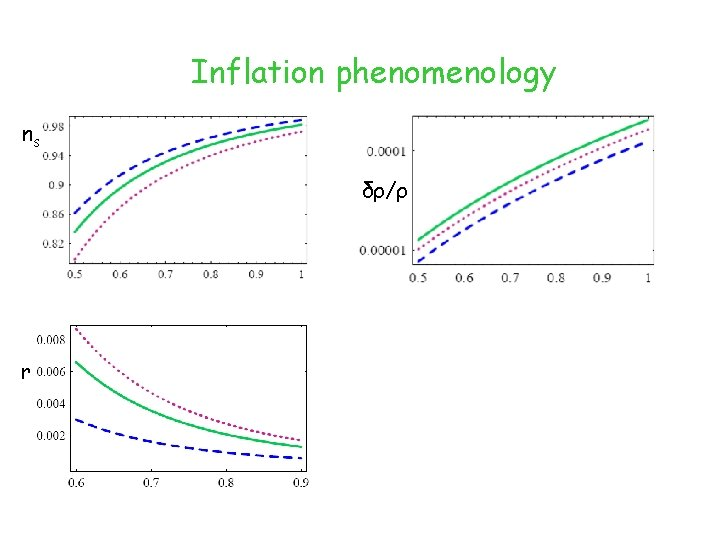Inflation phenomenology ns δρ/ρ r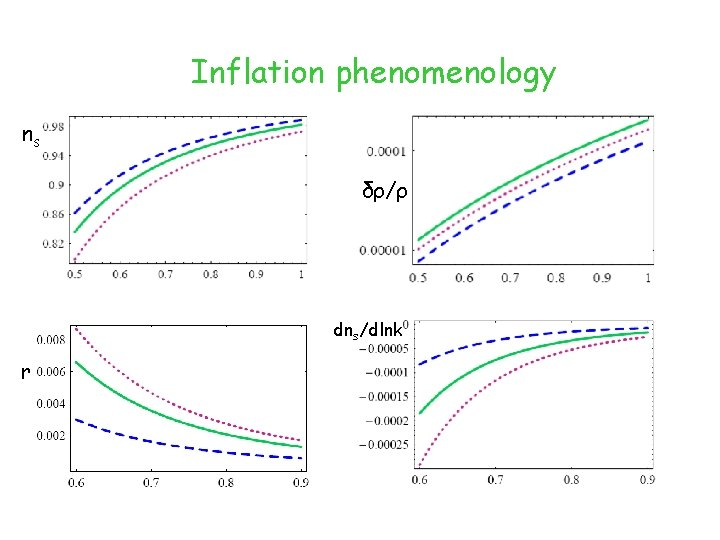Inflation phenomenology ns δρ/ρ dns/dlnk rConclusions A theory ENTIRELY written in terms of neutrino degrees of freedom is equivalent to a theory containing Φ. The resulting model is phenomenogically tighly constrained and can be (dis) probed in the near future. The model with more neutrinos is EVEN more beautiful (if such a thing is possible).